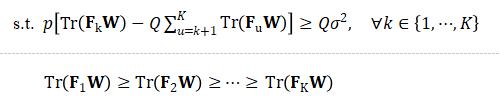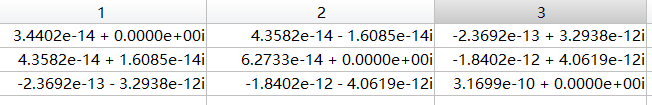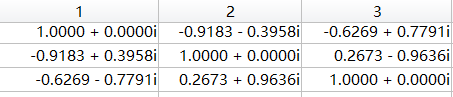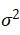# How to scale parameters correctly?

This is my contraint:F_k is a hermitian semidefinite matrix, the value of F_k is very small(as shown in illustration).the value of F_k is too small so that the cvx can’t solve the problem correctly, I don’t know how to scale the value correctly because the every value of F_k has different orders of magnitude.

Multiplying everything by 1e12 might be good. That would make all the non-zero elements in the illustration matrix be within a couple of orders of magnitude of one.

However, if other input matrices are significantly different than the illustration, there might be difficulties, and you would need to show us more information.

I tried to multiply everything by 1e12, but it doesn’t work. The W is a hermitian semidefinite matrix(as shown in illustration).the p is a scalar whose value is 0.1995, theis a scalar whose value is 3.9811e-15, the Q is a scalar whose value is 10.

Multiply all the F_i by 1e12.

Divide both sides of the first set of constraints by p, which makes the RHS be Q\sigma^2/p, and multiply that by 1e12, which makes the RHS 0.19955388471178, which equals 1e12*(10*3.9811e-15/0.1995)

You should check to make sure I didn’t make any mistakes. You want a mathematically equivalent problem, but with improved scaling.

You haven’t shown us what the other F_k look like, so I don’t know how well the 1e12 scaling will work. If the F_k are many orders of magnitude different than each other, it may be that double precision solvers can’[t reliably handle the problem. Higher precision solvers are available, but not under CVX.